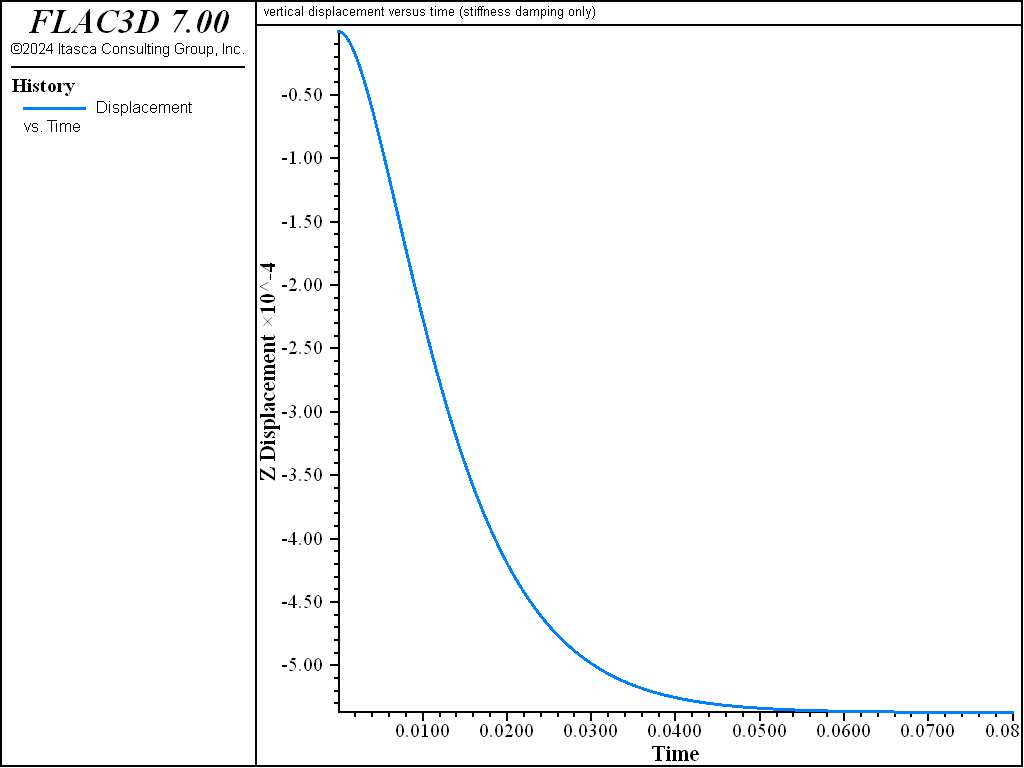# Block Under Gravity — Examining Damping Options

Note

To view this project in FLAC3D, use the menu command Help ‣ Examples…. Choose “Dynamic/MechanicalDamping” and select “MechanicalDamping.prj” to load. The main data files used are shown at the end of this example. The remaining data files can be found in the project.

In order to demonstrate how Rayleigh and Local damping works in FLAC3D, the results of several damping cases can be compared. The example consists of a square grid in which gravity is suddenly applied. The conditions are:

1. undamped;
2. Rayleigh damping (both mass and stiffness damping);
3. Rayleigh mass damping only;
4. Rayleigh stiffness damping only;
5. 5% Rayleigh damping; and
6. local damping.

In case (a), with no damping, a natural frequency of oscillation of approximately 25 Hertz is observed (see Figure 1). The problem should be critically damped using Rayleigh damping if: 1) a fraction of critical damping, $$\xi_{\min}$$, of 1 is specified; 2) the natural frequency of oscillation, $$f_{\min}$$, of 25 Hertz is specified; and 3) both mass and stiffness damping are used.Figure 1: Plot of vertical displacement versus time for gravity suddenly applied to a square grid (no damping).

The results in Figure 2 show that the problem is critically damped. If only mass or stiffness damping is used, then $$\xi_{\min}$$ must be doubled to obtain critical damping (since each component contributes one-half to the overall damping). Figure 3 and Figure 4 again show that the system is critically damped.

Note that the timestep is different for the three damped simulations. This is a result of the influence of stiffness-proportional damping, as discussed in Rayleigh Damping.Figure 2: Plot of vertical displacement versus time for gravity suddenly applied to a square grid (mass and stiffness damping).Figure 3: Plot of vertical displacement versus time for gravity suddenly applied to a square grid (mass damping only).Figure 4: Plot of vertical displacement versus time for gravity suddenly applied to a square grid (stiffness damping only).

Local damping contains some characteristics that make it attractive for dynamic simulations (see Local Damping for Dynamic Simulations). For the purpose of comparison, the example is run with 5% Rayleigh damping and compared to a result using a local damping coefficient calculated to match it. Displacement histories are given in Figures 5 and 6. The results are quite similar.

CAUTION: Local damping appears to give good results for a simple case because it is frequency-independent and needs no estimate of the natural frequency of the system being modeled. However, this type of damping should be treated with caution, and the results compared to those with Rayleigh damping for each application. There is some evidence to suggest that, for complicated waveforms, local damping underdamps the high frequency components and may introduce high frequency “noise.” Also note that local damping is timestep-dependent. Reducing the timestep will reduce the amount of energy removed from the system.

Local damping is not recommended for seismic simulations, because this type of damping cannot completely represent the energy loss of multiple cyclic loading properly.

Data File

;---------------------------------------------------------------------
;  Block under gravity - undamped and 3 critically damped cases
;---------------------------------------------------------------------
model new
model large-strain off
fish automatic-create off
model configure dynamic
; Create zones
zone create brick size 3,3,3
; Assign material model and properties
zone cmodel assign elastic
zone property bulk 1e8 shear 0.3e8 density 1000
; Initial conditions
model gravity 10
; Boundary conditions
zone gridpoint fix velocity-z range position-z 0
; Histories
history interval 1
zone history name='zdisp' displacement-z position 3.0,2.0,3.0
model history name='time' dynamic time-total
; Save common initial state
model save 'Initial'

; Run undamped state example
model restore 'Initial'
model title "vertical displacement versus time (undamped)"
model cycle 350
model save 'Undamped'

; Rayleigh mass + stiffness damping
model restore 'Initial'
model title "vertical displacement versus time (mass & stiffness damping)"
zone dynamic damping rayleigh 1 22.8
model solve time-total=0.08
model save 'RayleighMassStiff'

; Rayleigh mass only
model restore 'Initial'
model title "vertical displacement versus time (mass damping only)"
zone dynamic damping rayleigh 2 22.8 mass
model solve time-total=0.08
model save 'RayleighMass'

; Rayleigh stiff only
model restore 'Initial'
model title "vertical displacement versus time (stiffness damping only)"
zone dynamic damping rayleigh 2 22.8 stiffness
model solve time-total=0.08
model save 'RayleighStiff'

; 5% Ralyeigh damping
model restore 'Initial'
model title "vertical displacement versus time (5% Rayleigh damping)"
zone dynamic damping rayleigh 0.05 22.8
history interval=5
model solve time-total=0.5
model save 'Rayleigh05'

; Local damping
model restore 'Initial'
model title "vertical displacement versus time (5% Local damping)"
zone dynamic damping local 0.1571 ; = math.pi * 0.05
history interval=5
model solve time-total=0.5
model save 'Local'